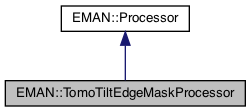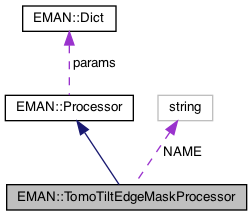EMAN2

A processor designed specifically for tomographic tilt series data. More...

`#include <processor.h>`[legend][legend]

## Classes

class  GaussianFunctoid

## Public Member Functions

virtual void process_inplace (EMData *image)
To process an image in-place. More...

virtual string get_name () const
Get the processor's name. More...

virtual TypeDict get_param_types () const
Get processor parameter information in a dictionary. More...

virtual string get_desc () const
Get the descrition of this specific processor. More...Public Member Functions inherited from EMAN::Processor
virtual ~Processor ()

virtual EMDataprocess (const EMData *const image)
To proccess an image out-of-place. More...

virtual void process_list_inplace (vector< EMData * > &images)
To process multiple images using the same algorithm. More...

virtual Dict get_params () const
Get the processor parameters in a key/value dictionary. More...

virtual void set_params (const Dict &new_params)
Set the processor parameters using a key/value dictionary. More...

## Static Public Member Functions

static ProcessorNEW ()Static Public Member Functions inherited from EMAN::Processor
static string get_group_desc ()
Get the description of this group of processors. More...

static void EMFourierFilterInPlace (EMData *fimage, Dict params)
Compute a Fourier-filter processed image in place. More...

static EMDataEMFourierFilter (EMData *fimage, Dict params)
Compute a Fourier-processor processed image without altering the original image. More...

## Static Public Attributes

static const string NAME = "tomo.tiltedgemask"Public Types inherited from EMAN::Processor
enum  fourier_filter_types {
TOP_HAT_LOW_PASS , TOP_HAT_HIGH_PASS , TOP_HAT_BAND_PASS , TOP_HOMOMORPHIC ,
GAUSS_LOW_PASS , GAUSS_HIGH_PASS , GAUSS_BAND_PASS , GAUSS_INVERSE ,
GAUSS_HOMOMORPHIC , BUTTERWORTH_LOW_PASS , BUTTERWORTH_HIGH_PASS , BUTTERWORTH_HOMOMORPHIC ,
KAISER_I0 , KAISER_SINH , KAISER_I0_INVERSE , KAISER_SINH_INVERSE ,
SHIFT , TANH_LOW_PASS , TANH_HIGH_PASS , TANH_HOMOMORPHIC ,
}
Fourier filter Processor type enum. More...Protected Attributes inherited from EMAN::Processor
Dict params

## Detailed Description

A processor designed specifically for tomographic tilt series data.

This processors masks out 'mass' in tilted images that is not present in the zero-tilt (0 degrees) image. It does this based on the tilt angle. The tilt angle can be extracted from the image metadata (stored as the euler_alt attribute), or it may be specified explicitly (specifying the angle is the default behavior). The masked out regions at both sides of the image are set to 0 by default, but can also be set to the mean of the nearest non-masked data edge (in the y direction), or similarly the mean of both non-masked data edges on either side of the image. A gaussian fall-off is optional (but off by default).

Date
01/10/2008
Parameters
 biedgemean Mutually exclusive of edgemean. Experimental. Causes the pixels in the masked out areas to take the average value of both the left and right edge pixel strips edgemean Mutually exclusive of biedgemean. Masked pixels values assume the mean edge pixel value, independently, for both sides of the image angle The angle that the image is, with respect to the zero tilt image angle_fim Read fim as 'from image metadata' - this causes the altitude angle stored in by the image object (i.e. as extracted from the header, as currently stored in memory) to be used as the angle. This overrides the angle argument gauss_falloff Causes the edge masking to have a smooth Gaussian fall-off - this parameter specifies how many pixels the fall-off will proceed over. Default is 0 gauss_sigma The sigma of the Gaussian function used to smooth the edge fall-off (functional form is exp(-(pixel distance)^2/sigma^2)

Definition at line 9005 of file processor.h.

## ◆ get_desc()

 virtual string EMAN::TomoTiltEdgeMaskProcessor::get_desc ( ) const
inlinevirtual

Get the descrition of this specific processor.

This function must be overwritten by a subclass.

Returns
The description of this processor.

Implements EMAN::Processor.

Definition at line 9032 of file processor.h.

9033 {
9034 return "Masks the part of the image which is not present in the 0-tilt image. Masked areas can be 0 or set to the edgemean (of the nearest or both edges). Masked areas can also have a Gaussian fall-off to make the appearance smooth.";
9035 }

## ◆ get_name()

 virtual string EMAN::TomoTiltEdgeMaskProcessor::get_name ( ) const
inlinevirtual

Get the processor's name.

Each processor is identified by a unique name.

Returns
The processor's name.

Implements EMAN::Processor.

Definition at line 9010 of file processor.h.

9011 {
9012 return NAME;
9013 }
static const string NAME
Definition: processor.h:9037

References NAME.

## ◆ get_param_types()

 virtual TypeDict EMAN::TomoTiltEdgeMaskProcessor::get_param_types ( ) const
inlinevirtual

Get processor parameter information in a dictionary.

Each parameter has one record in the dictionary. Each record contains its name, data-type, and description.

Returns
A dictionary containing the parameter info.

Reimplemented from EMAN::Processor.

Definition at line 9020 of file processor.h.

9021 {
9022 TypeDict d;
9023 d.put("biedgemean", EMObject::BOOL, "Mutually exclusive of edgemean. Experimental. Causes the pixels in the masked out areas to take the average value of both the left and right edge pixel strips");
9024 d.put("edgemean", EMObject::BOOL, "Mutually exclusive of biedgemean. Masked pixels values assume the mean edge pixel value, independently, for both sides of the image.");
9025 d.put("angle", EMObject::INT, "The angle that the image is, with respect to the zero tilt image");
9026 d.put("gauss_falloff",EMObject::INT, "Causes the edge masking to have a smooth Gaussian fall-off - this parameter specifies how many pixels the fall-off will proceed over. Default is 0.");
9027 d.put("gauss_sigma",EMObject::FLOAT,"The sigma of the Gaussian function used to smooth the edge fall-off (functional form is exp(-(pixel distance)^2/sigma^2)");
9028 d.put("angle_fim",EMObject::BOOL,"Read fim as 'from image metadata' - this causes the altitude angle stored in by the image object (i.e. as extracted from the header, as currently stored in memory) to be used as the angle. This overrides the angle argument");
9029 return d;
9030 }
TypeDict is a dictionary to store <string, EMObject::ObjectType> pair.
Definition: emobject.h:305
void put(const string &key, EMObject::ObjectType o, const string &desc="")
Definition: emobject.h:330

## ◆ NEW()

 static Processor * EMAN::TomoTiltEdgeMaskProcessor::NEW ( )
inlinestatic

Definition at line 9015 of file processor.h.

9016 {
9018 }
A processor designed specifically for tomographic tilt series data.
Definition: processor.h:9006

## ◆ process_inplace()

 void TomoTiltEdgeMaskProcessor::process_inplace ( EMData * image )
virtual

To process an image in-place.

For those processors which can only be processed out-of-place, override this function to just print out some error message to remind user call the out-of-place version.

Parameters
 image The image to be processed.

Implements EMAN::Processor.

Definition at line 12776 of file processor.cpp.

12777{
12778 bool biedgemean = params.set_default("biedgemean", false);
12779 bool edgemean = params.set_default("edgemean", false);
12780 // You can only do one of these - so if someone specifies them both the code complains loudly
12781 if (biedgemean && edgemean) throw InvalidParameterException("The edgemean and biedgemean options are mutually exclusive");
12782
12783 bool fim = params.set_default("angle_fim", false);
12784 float alt;
12785 if ( fim ) {
12786 Transform* t = (Transform*)image->get_attr("xform.projection");
12787 Dict d = t->get_params("eman");
12788 alt = (float) d["alt"];
12789 if(t) {delete t; t=0;}
12790 }
12791 else alt = params.set_default("angle", 0.0f);
12792
12793
12794 float cosine = cos(alt*M_PI/180.0f);
12795
12796 // Zero the edges
12797 int nx = image->get_xsize();
12798 int ny = image->get_ysize();
12799 int x_clip = static_cast<int>( (float) nx * ( 1.0 - cosine ) / 2.0);
12800
12801 float x1_edge_mean = 0.0;
12802 float x2_edge_mean = 0.0;
12803
12804 if ( biedgemean )
12805 {
12806 float edge_mean = 0.0;
12807
12808 // Accrue the pixel densities on the side strips
12809 for ( int i = 0; i < ny; ++i ) {
12810 edge_mean += image->get_value_at(x_clip, i );
12811 edge_mean += image->get_value_at(nx - x_clip-1, i );
12812 }
12813 // Now make it so the mean is stored
12814 edge_mean /= 2*ny;
12815
12816 // Now shift pixel values accordingly
12817 for ( int i = 0; i < ny; ++i ) {
12818 for ( int j = nx-1; j >= nx - x_clip; --j) {
12819 image->set_value_at(j,i,edge_mean);
12820 }
12821 for ( int j = 0; j < x_clip; ++j) {
12822 image->set_value_at(j,i,edge_mean);
12823 }
12824 }
12825 x1_edge_mean = edge_mean;
12826 x2_edge_mean = edge_mean;
12827 }
12828 else if (edgemean)
12829 {
12830 for ( int i = 0; i < ny; ++i ) {
12831 x1_edge_mean += image->get_value_at(x_clip, i );
12832 x2_edge_mean += image->get_value_at(nx - x_clip-1, i );
12833 }
12834 x1_edge_mean /= ny;
12835 x2_edge_mean /= ny;
12836
12837 for ( int i = 0; i < ny; ++i ) {
12838 for ( int j = 0; j < x_clip; ++j) {
12839 image->set_value_at(j,i,x1_edge_mean);
12840 }
12841 for ( int j = nx-1; j >= nx - x_clip; --j) {
12842 image->set_value_at(j,i,x2_edge_mean);
12843 }
12844 }
12845 }
12846 else
12847 {
12848 // The edges are just zeroed -
12849 Dict zero_dict;
12850 zero_dict["x0"] = x_clip;
12851 zero_dict["x1"] = x_clip;
12852 zero_dict["y0"] = 0;
12853 zero_dict["y1"] = 0;
12855 }
12856
12857 int gauss_rad = params.set_default("gauss_falloff", 0);
12858 if ( gauss_rad != 0)
12859 {
12860 // If the gaussian falloff distance is greater than x_clip, it will technically
12861 // go beyond the image boundaries. Thus we clamp gauss_rad so this cannot happen.
12862 // Therefore, there is potential here for (benevolent) unexpected behavior.
12864
12865 float gauss_sigma = params.set_default("gauss_sigma", 3.0f);
12866 if ( gauss_sigma < 0 ) throw InvalidParameterException("Error - you must specify a positive, non-zero gauss_sigma");
12867 float sigma = (float) gauss_rad/gauss_sigma;
12868
12869 GaussianFunctoid gf(sigma);
12870
12871 for ( int i = 0; i < ny; ++i ) {
12872
12873 float left_value = image->get_value_at(x_clip, i );
12874 float scale1 = left_value-x1_edge_mean;
12875
12876 float right_value = image->get_value_at(nx - x_clip - 1, i );
12877 float scale2 = right_value-x2_edge_mean;
12878
12879 for ( int j = 1; j < gauss_rad; ++j )
12880 {
12881 image->set_value_at(x_clip-j, i, scale1*gf((float)j)+x1_edge_mean );
12882 image->set_value_at(nx - x_clip + j-1, i, scale2*gf((float)j)+x2_edge_mean);
12883 }
12884 }
12885 }
12886
12887 image->update();
12888}
Dict is a dictionary to store <string, EMObject> pair.
Definition: emobject.h:385
type set_default(const string &key, type val)
Default setting behavior This can be achieved using a template - d.woolford Jan 2008 (before there wa...
Definition: emobject.h:569
A Transform object is a somewhat specialized object designed specifically for EMAN2/Sparx storage of ...
Definition: transform.h:75
Dict get_params(const string &euler_type) const
Get the parameters of the entire transform, using a specific euler convention.
Definition: transform.cpp:479
#define InvalidParameterException(desc)
Definition: exception.h:361